# 常见的激活函数

``````# 初始化一些信息
import torch
import torch.nn.functional as F
import matplotlib.pyplot as plt
import numpy as np
x= torch.linspace(-10,10,60)
``````

## Sigmoid 函数

``````g(z) = a = 1 / (1 + e^(-z))
g'(z) = a' = a (1 - a)
``````

``````ax = plt.gca()
ax.spines['right'].set_color('none')
ax.spines['top'].set_color('none')
ax.xaxis.set_ticks_position('bottom')
ax.spines['bottom'].set_position(('data', 0))
ax.yaxis.set_ticks_position('left')
ax.spines['left'].set_position(('data', 0))
plt.ylim((0, 1))
sigmod=torch.sigmoid(x)
plt.plot(x.numpy(),sigmod.numpy())
``````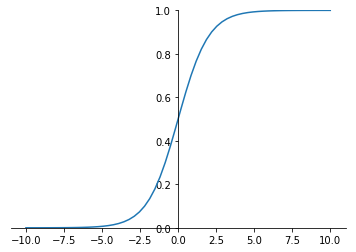## Tanh 函数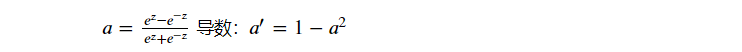tanh是双曲正切函数，输出区间是在(-1,1)之间，而且整个函数是以0为中心

``````ax = plt.gca()
ax.spines['right'].set_color('none')
ax.spines['top'].set_color('none')
ax.xaxis.set_ticks_position('bottom')
ax.spines['bottom'].set_position(('data', 0))
ax.yaxis.set_ticks_position('left')
ax.spines['left'].set_position(('data', 0))
plt.ylim((-1, 1))
tanh=torch.tanh(x)
plt.plot(x.numpy(),tanh.numpy())
``````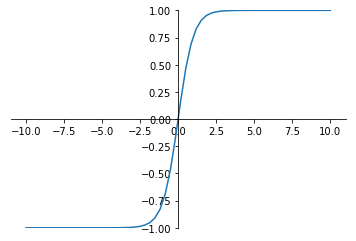## ReLu 函数

Relu(Rectified Linear Units) 修正线性单元

``````a = max(0, z)
``````
• 当 z > 0 时，梯度始终为1，从而提高神经网络基于梯度算法的运算速度。
• 当 z < 0 时，梯度始终为0

ReLu函数只有线性关系（只需要判断输入是否大于0），不管是前向传播还是反向传播，都比Simoid和Tanh要快很多

``````ax = plt.gca()
ax.spines['rights'].set_color('none')
ax.spines['top'].set_color('none')
ax.xaxis.set_ticks_position('bottom')
ax.spines['bottom'].set_position(('data', 0))
ax.yaxis.set_ticks_position(('data', 0))
plt.ylim((-3, 10))
relu = F.relu(x)
plt.plot(x.numpy(), relu.numpy())
``````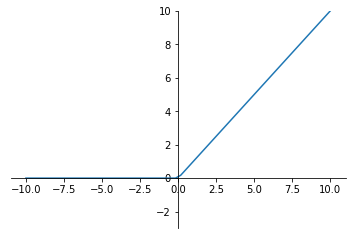## Leaky Relu 函数

ReLU的前半段设为αz而非0，通常α=0.01

``````a = max(𝛼z, z)
``````
``````ax = plt.gca()
ax.spines['right'].set_color('none')
ax.spines['top'].set_color('none')
ax.xaxis.set_ticks_position('bottom')
ax.spines['bottom'].set_position(('data', 0))
ax.yaxis.set_ticks_position('left')
ax.spines['left'].set_position(('data', 0))
plt.ylim((-3, 10))
l_relu=F.leaky_relu(x,0.1) # 这里的0.1是为了方便展示，理论上应为0.01甚至更小的值
plt.plot(x.numpy(),l_relu.numpy())
``````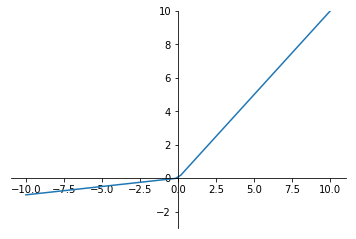ReLU目前仍是最常用的activation function，在隐藏层中推荐优先尝试！